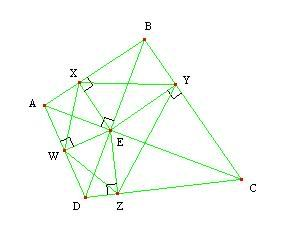## Thursday, April 13, 2006

### Cyclicity. Topic: Geometry. Level: Olympiad.

Problem: (1993 USAMO - #2) Let $ABCD$ be a convex quadrilateral such that diagonals $AC$ and $BD$ intersect at right angles, and let $E$ be their intersection. Prove that the reflections of $E$ across $AB, BC, CD, DA$ are concyclic.Solution: Let $X,Y,Z,W$ be the feet of the perpendiculars from $E$ to $AB, BC, CD, DA$, respectively. Since the reflections of $E$ across the sides are on lines $EX, EY, EZ, EW$, just twice as far, we have $XYZW$ similar to the quadrilateral formed by the reflections. Hence it is sufficient to show that $XYZW$ is cyclic.

Consider quadrilaterals $EWAX, EXBY, EYCZ, EZCW$. They are all cyclic because of the right angles formed at $X,Y,Z,W$ (as shown in the diagram).

Thus we have $\angle XWE = \angle XAE$, $\angle ZWE = \angle ZDE$, $\angle XYE = \angle XBE$, and $\angle ZYE = \angle ZCE$.

Therefore, we have

$\angle XWZ = \angle XWE + \angle ZWE = \angle XAE + \angle ZDE$

$\angle XYZ = \angle XYE + \angle ZYE = \angle XBE + \angle ZCE$.

Also note that

$\angle XAE + \angle XBE = 90^{\circ}$ and $\angle ZDE + \angle ZCE = 90^{\circ}$ (1)

because the diagonals are perpendicular.

Finally,

$\angle XWZ + \angle XYZ = (\angle XAE+\angle XBE)+(\angle ZDE+\angle ZCE) = 180^{\circ}$

by (1), which means $XYZW$ is cyclic, as desired. QED.

--------------------

Comment: A decent amount of angle-chasing in this problem, but working it out wasn't bad. When you need to write all the steps in a proof, it can look a little messy and a little easy to get lost.

--------------------

Practice Problem: Given finitely many points in a plane, it is known that the area of the triangle formed by any three points of the set is less than $1$. Show that all points of the set lie inside or on boundary of a triangle with area less than $4$.